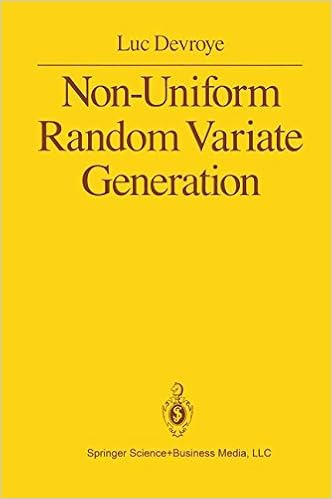## ratio-of-uniforms [#4]Possibly the last post on random number generation by Kinderman and Monahan’s (1977) ratio-of-uniform method. After fiddling with the Gamma(a,1) distribution when a<1 for a while, I indeed figured out a way to produce a bounded set with this method: considering an arbitrary cdf Φ with corresponding pdf φ, the uniform distribution on the set Λ of (u,v)’s in R⁺xX such that

0≤u≤Φοƒ[φοΦ⁻¹(u)v]

induces the distribution with density proportional to ƒ on φοΦ⁻¹(U)V. This set Λ has a boundary that is parameterised as

u=Φοƒ(x),  v=1/φοƒ(x), x∈Χ

which remains bounded in u since Φ is a cdf and in v if φ has fat enough tails. At both 0 and ∞. When ƒ is the Gamma(a,1) density this can be achieved if φ behaves like log(x)² near zero and like a inverse power at infinity. Without getting into all the gory details, closed form density φ and cdf Φ can be constructed for all a’s, as shown for a=½ by the boundaries in u and v (yellow) belowwhich leads to a bounded associated set ΛAt this stage, I remain uncertain of the relevance of such derivations, if only because the set A thus derived is ill-suited for uniform draws proposed on the enclosing square box. And also because a Gamma(a,1) simulation can rather simply be derived from a Gamma(a+1,1) simulation. But, who knows?!, there may be alternative usages of this representation, such as innovative slice samplers. Which means the ratio-of-uniform method may reappear on the ‘Og one of those days…

This site uses Akismet to reduce spam. Learn how your comment data is processed.